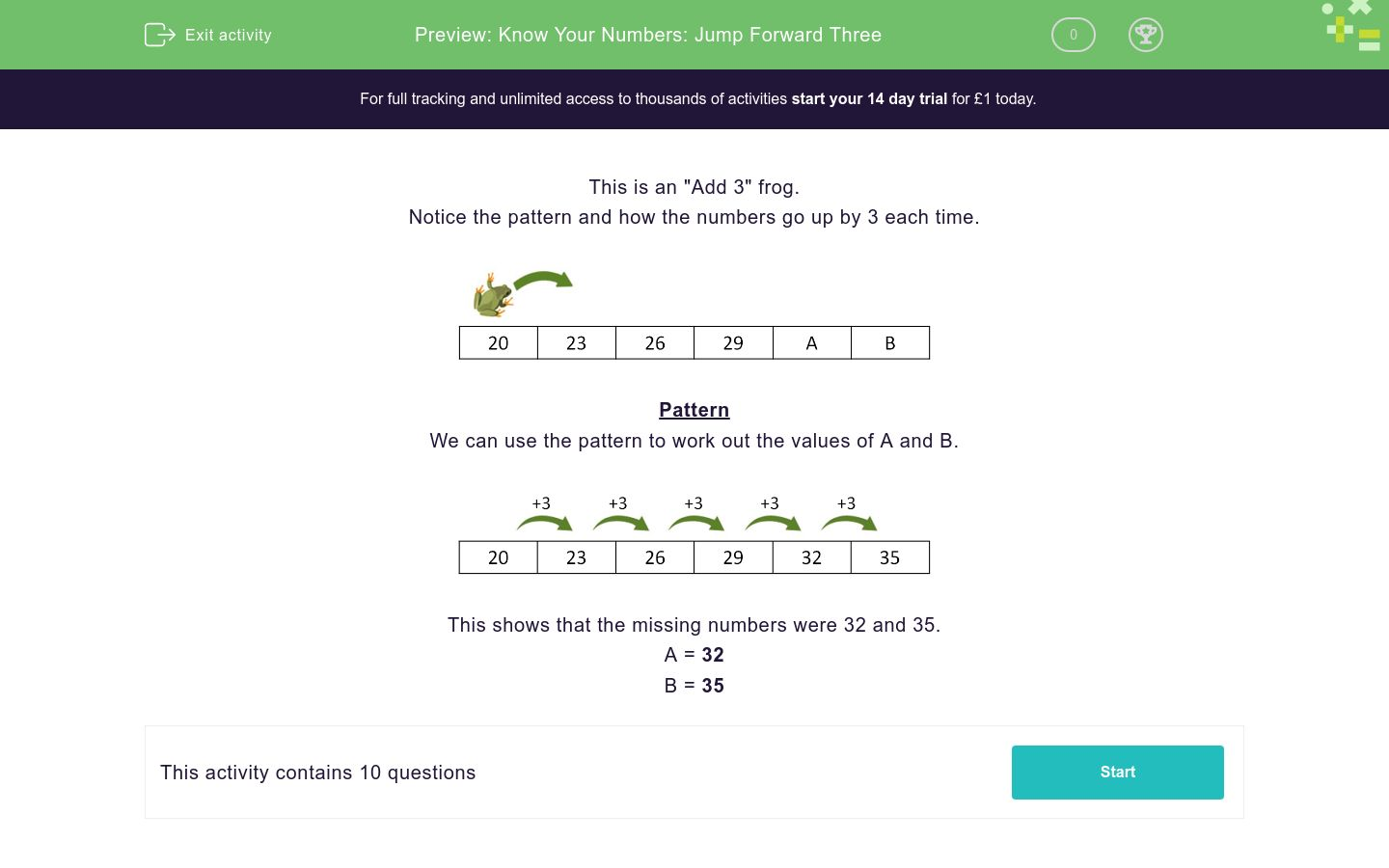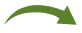# Know Your Numbers: Jump Forward Three

In this worksheet, students find the missing numbers in a sequence that goes up in threes.Key stage:  KS 1

Curriculum topic:   Number: Number and Place Value

Curriculum subtopic:   Count in Steps (2, 3, 5 and 10)

Difficulty level:### QUESTION 1 of 10

This is an "Add 3" frog.

Notice the pattern and how the numbers go up by 3 each time.Pattern

We can use the pattern to work out the values of A and B.This shows that the missing numbers were 32 and 35.

A = 32

B = 35

This is an "Add 2" frog.

Work out the values of A and B.16 18 20 22 A B
 A B 24 28 22 26

This is an "Add 3" frog.

Work out the values of A and B.11 A 17 20 B 26
 A B 13 14 22 23

This is an "Add 3" frog.

Work out the values of A and B.10 A 16 19 B 25
 A B 12 13 21 22

This is an "Add 3" frog.

Work out the values of A and B.21 24 A 30 B 36
 A B 32 24 27 33

This is an "Add 3" frog.

Work out the values of A and B.14 17 A B 26 29
 A B 32 28 20 23

This is an "Add 3" frog.

Work out the values of A and B.15 18 A 24 27 B
 A B 30 23 21 31

This is an "Add 3" frog.

Work out the values of A and B.7 10 A 16 19 B
 A B 13 23 24 22

This is an "Add 3" frog.

Work out the values of A and B.17 20 A 26 29 B
 A B 23 22 24 32

This is an "Add 3" frog.

Work out the values of A and B.13 16 A 22 25 B
 A B 17 19 27 28

This is an "Add 3" frog.

Work out the values of A and B.A 4 B 10 13 16
 A B 1 9 7 8
• Question 1

This is an "Add 2" frog.

Work out the values of A and B.16 18 20 22 A B
 A B 24 28 22 26
EDDIE SAYS

Pattern:

 +2+2+2+2+216 18 20 22 24 26
• Question 2

This is an "Add 3" frog.

Work out the values of A and B.11 A 17 20 B 26
 A B 13 14 22 23
• Question 3

This is an "Add 3" frog.

Work out the values of A and B.10 A 16 19 B 25
 A B 12 13 21 22
• Question 4

This is an "Add 3" frog.

Work out the values of A and B.21 24 A 30 B 36
 A B 32 24 27 33
• Question 5

This is an "Add 3" frog.

Work out the values of A and B.14 17 A B 26 29
 A B 32 28 20 23
• Question 6

This is an "Add 3" frog.

Work out the values of A and B.15 18 A 24 27 B
 A B 30 23 21 31
• Question 7

This is an "Add 3" frog.

Work out the values of A and B.7 10 A 16 19 B
 A B 13 23 24 22
• Question 8

This is an "Add 3" frog.

Work out the values of A and B.17 20 A 26 29 B
 A B 23 22 24 32
• Question 9

This is an "Add 3" frog.

Work out the values of A and B.13 16 A 22 25 B
 A B 17 19 27 28
• Question 10

This is an "Add 3" frog.

Work out the values of A and B.A 4 B 10 13 16
 A B 1 9 7 8
---- OR ----

Sign up for a £1 trial so you can track and measure your child's progress on this activity.

### What is EdPlace?

We're your National Curriculum aligned online education content provider helping each child succeed in English, maths and science from year 1 to GCSE. With an EdPlace account you’ll be able to track and measure progress, helping each child achieve their best. We build confidence and attainment by personalising each child’s learning at a level that suits them.

Get started